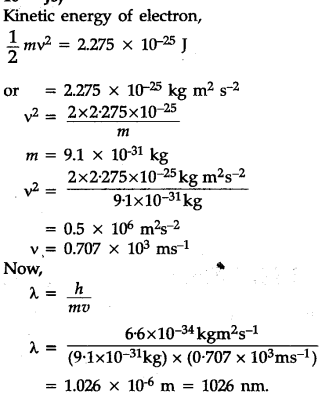# An electron is moving with a kinetic energy of 2.275 X \${{10}^{-25}}\$ J. Calculate its de-Broglie wavelength. (Mass of electron = 9.1 x \${{10}^{-31}}\$ kg, h = 6.6 x \${{10}^{-34}}\$ Js